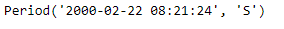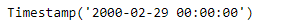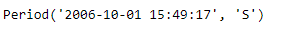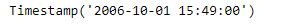Python | Pandas Period.to_timestamp

• Last Updated : 06 Jan, 2019

Python is a great language for doing data analysis, primarily because of the fantastic ecosystem of data-centric python packages. Pandas is one of those packages and makes importing and analyzing data much easier.

Pandas Period.to_timestamp() function return the Timestamp representation of the Period at the target frequency at the specified end (how) of the Period.

Syntax : Period.to_timestamp()

Parameters :
freq : Target frequency. Default is ‘D’ if self.freq is week or longer and ‘S’ otherwise
how : ‘S’, ‘E’. Can be aliased as case insensitive ‘Start’, ‘Finish’, ‘Begin’, ‘End’

Return : Timestamp

Example #1: Use Period.to_timestamp() function to return the given period object as a Timestamp object in the specified frequency.

 # importing pandas as pdimport pandas as pd  # Create the Period objectprd = pd.Period(freq ='S', year = 2000, month = 2, day = 22,                         hour = 8, minute = 21, second = 24)  # Print the Period objectprint(prd)

Output :Now we will use the Period.to_timestamp() function to return the given period object as a timestamp object.

 # return as a timestamp in the specified frequency.# 'M' represents monthly frequencyprd.to_timestamp(freq ='M')

Output :As we can see in the output, the Period.to_timestamp() function has returned the given period object as a timestamp in the specified frequency.

Example #2: Use Period.to_timestamp() function to return the given period object as a Timestamp object in the specified frequency.

 # importing pandas as pdimport pandas as pd  # Create the Period objectprd = pd.Period(freq ='S', year = 2006, month = 10,               hour = 15, minute = 49, second = 17)  # Print the objectprint(prd)

Output :Now we will use the Period.to_timestamp() function to return the given period object as a timestamp object.

 # return as a timestamp in the specified frequency.# 'T' represents minutely frequencyprd.to_timestamp(freq ='T')

Output :As we can see in the output, the Period.to_timestamp() function has returned the given period object as a timestamp in the specified frequency.

Attention geek! Strengthen your foundations with the Python Programming Foundation Course and learn the basics.

To begin with, your interview preparations Enhance your Data Structures concepts with the Python DS Course. And to begin with your Machine Learning Journey, join the Machine Learning - Basic Level Course

My Personal Notes arrow_drop_up